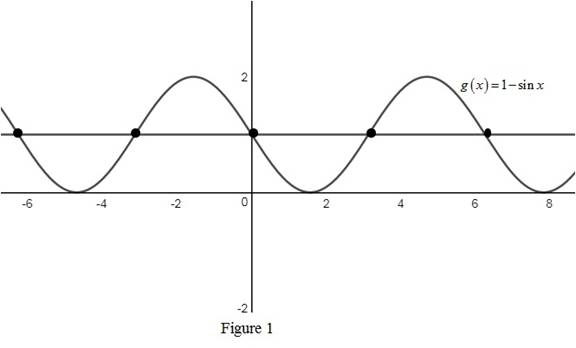# Whether the function g ( x ) = 1 − sin x is one-to-one.### Single Variable Calculus: Concepts...

4th Edition
James Stewart
Publisher: Cengage Learning
ISBN: 9781337687805### Single Variable Calculus: Concepts...

4th Edition
James Stewart
Publisher: Cengage Learning
ISBN: 9781337687805

#### Solutions

Chapter 1.6, Problem 11E
To determine

## Whether the function g(x)=1−sinx is one-to-one.

Expert Solution

The function g(x)=1sinx is not a one-to one function.

### Explanation of Solution

Result used:

Horizontal line test states that the graph of the function is one-to-one function if and only if a horizontal line intersects the graph exactly once.

Calculation:

Use the horizontal line test to determine whether the function g(x)=1sinx is one-to-one.

Sketch the graph of the function g(x)=1sinx and perform the horizontal line test as shown below in Figure 1.From Figure 1, it is observed that the horizontal line intersects the curve at many points, which means it fails the horizontal line test.

Therefore, the function g(x)=1sinx is not a one-to-one function.

### Have a homework question?

Subscribe to bartleby learn! Ask subject matter experts 30 homework questions each month. Plus, you’ll have access to millions of step-by-step textbook answers!